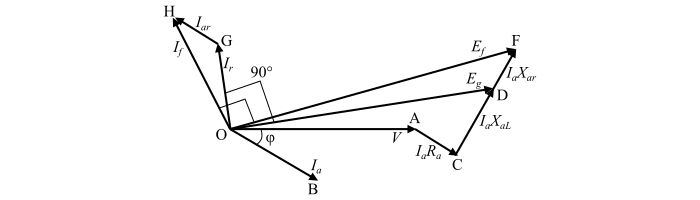# Potier Triangle Method ŌĆō Determining the Voltage Regulation of Alternators

The Potier Triangle Method is used in determining the voltage regulation of alternators. It is also known as the Zero Power Factor (ZPF) method. The following assumptions are made in the Potier triangle method

• The armature reaction MMF is constant.

• The open-circuit characteristic (O.C.C.) taken on no-load accurately represents the relation between MMF and voltage under loaded conditions.

• The voltage drop due to the armature leakage reactance ($­ØÉ╝_{­ØæÄ}­Øæŗ_{­ØæÄ­ØÉ┐}$) is independent of the excitation.

## Procedure to Obtain Voltage Regulation by ZPF Method

The following procedure is followed to determine the voltage regulation of an alternator or synchronous generator by the zero power factor (ZPF) method −

First of all, we draw the phasor diagram of the alternator at lagging power factor as shown in the figure.In the phasor diagram,

• The phasor OA represents the terminal phase voltage (V) at full-load. It is taken as the reference phasor, thus drawn horizontally.

• The phasor OB represents the full-load current ($­ØÉ╝_{­ØæÄ}$). Since, the load power factor is φ lagging, hence, it is drawn lagging behind the voltage (V) by the power factor angle (φ).

• The phasor AC is representing the voltage drop ($­ØÉ╝_{­ØæÄ}­Øæģ_{­ØæÄ}$) in the armature resistance. As it is in phase with the armature current ($­ØÉ╝_{­ØæÄ}$) and hence is drawn parallel to current phasor ($­ØÉ╝_{­ØæÄ}$). If the armature resistance is neglected, then, this phasor will not be drawn in the phasor diagram.

• The voltage drop due to leakage reactance ($­ØÉ╝_{­ØæÄ}­Øæŗ_{­ØæÄ­ØÉ┐}$) is represented by the phasor CD. It is perpendicular to the phasor AC.

• Now, join O and D, the phasor OD represents the generated EMF ($­ØÉĖ_{­Øæö}$).

Now, find the field excitation current ($­ØÉ╝_{­Øæ¤}$) of the resultant MMF which is corresponding to the generated EMF ($­ØÉĖ_{­Øæö}$) from the open-circuit characteristic (O.C.C.). Then, draw the phasor OG equal to the current ($­ØÉ╝_{­Øæ¤}$) and it is perpendicular to the phasor OD.

Draw the phasor GH parallel to the load current ($­ØÉ╝_{­ØæÄ}$) to represent the field current equivalent to the full-load armature reaction current ($­ØÉ╝_{a­Øæ¤}$). Therefore, the phasor OH gives the total field current ($­ØÉ╝_{­Øæō}$).

When the load on the alternator is thrown off, then the terminal voltage will be equal to the generated EMF, corresponding to the field current ($­ØÉ╝_{­Øæō}$ = ­Øæé­ØÉ╗).

Now, determine the EMF ($­ØÉĖ_{­Øæō}$) represented by the phasor OF corresponding to the field current ($­ØÉ╝_{­Øæō} = ­Øæé­ØÉ╗$) from the O.C.C. The phasor OF will lag behind the phasor OH by 90°. Also, the voltage drop due to the armature reaction is represented by the phasor DF.

Therefore, the voltage regulation of the alternator or synchronous generator can be obtained from the following expression −

$$\mathrm{\%\:voltage\:regulation =\frac{­ØÉĖ_{­Øæō} − ­Øæē}{­Øæē} \times 100}$$